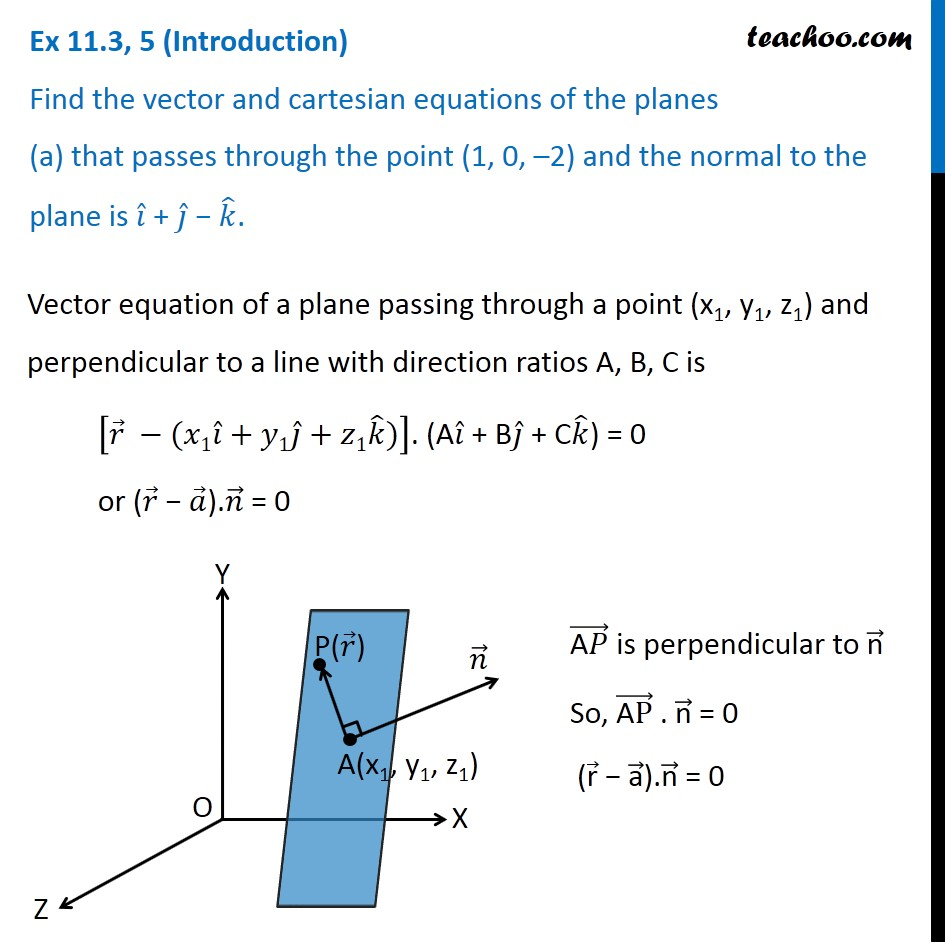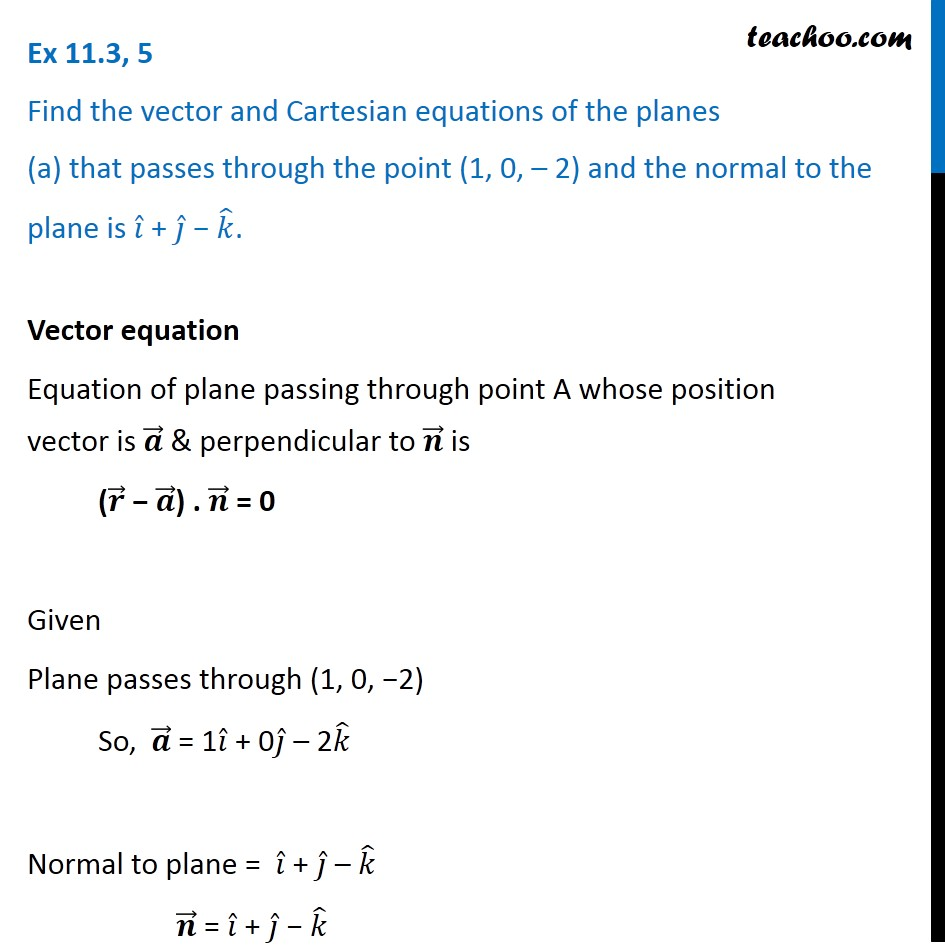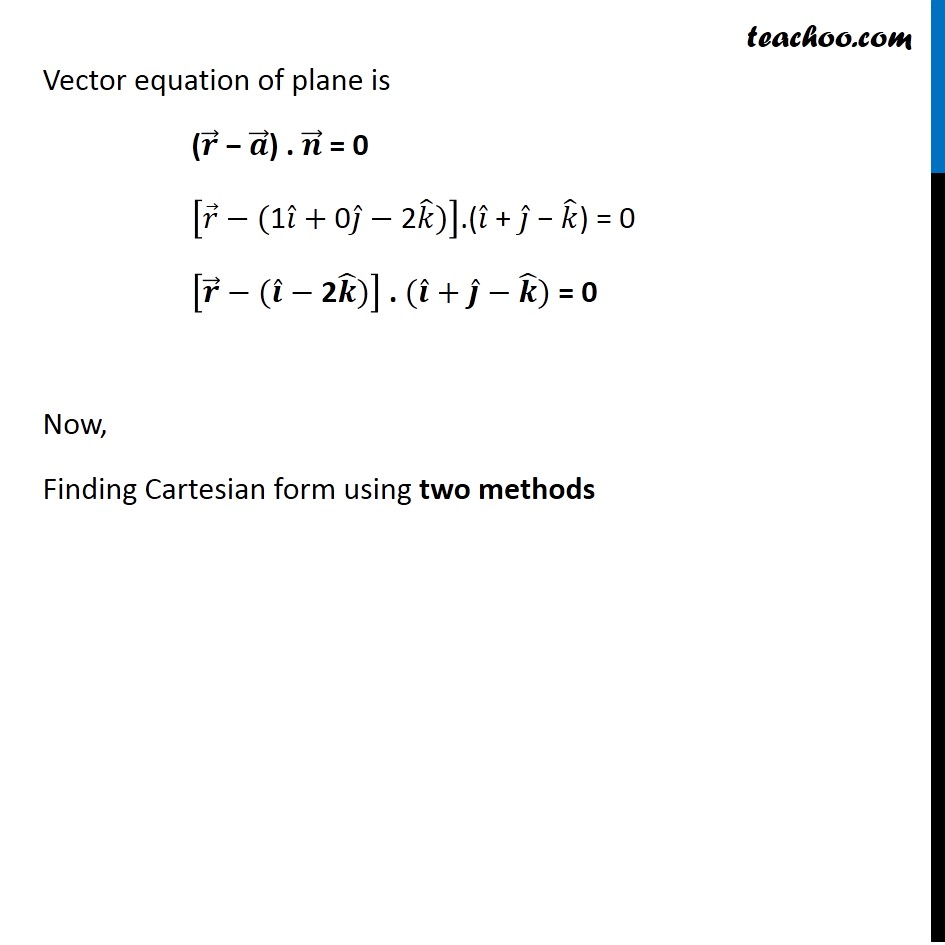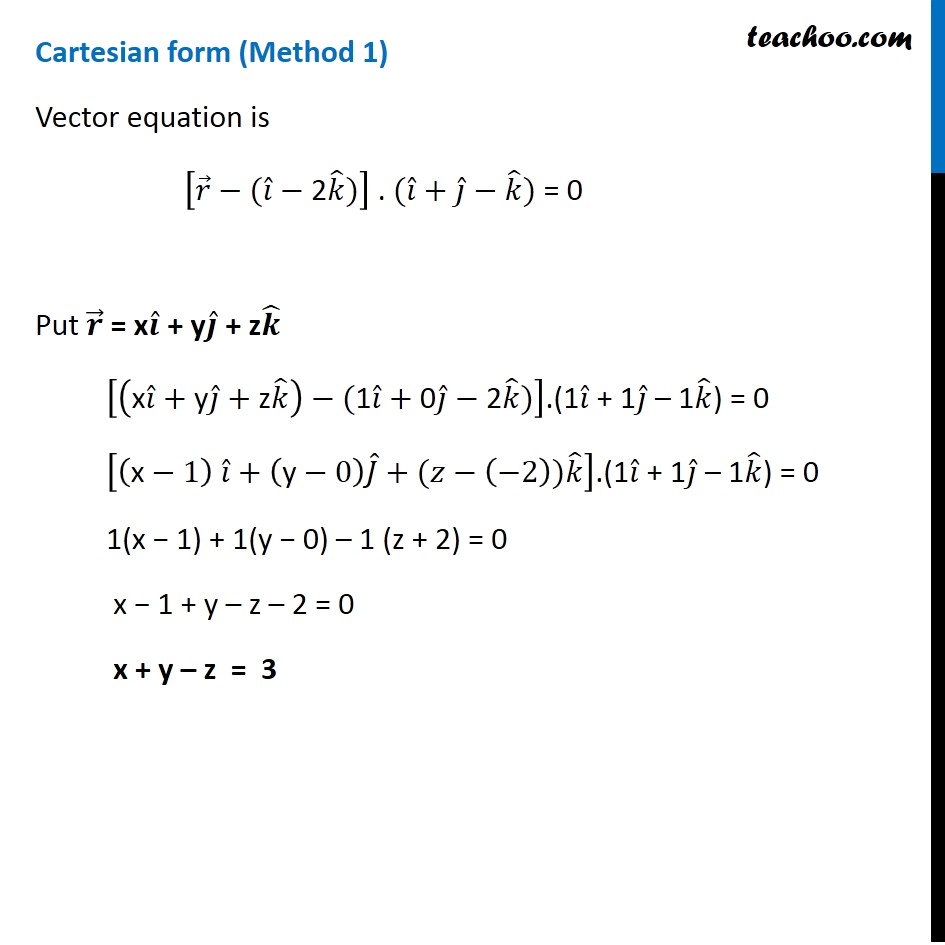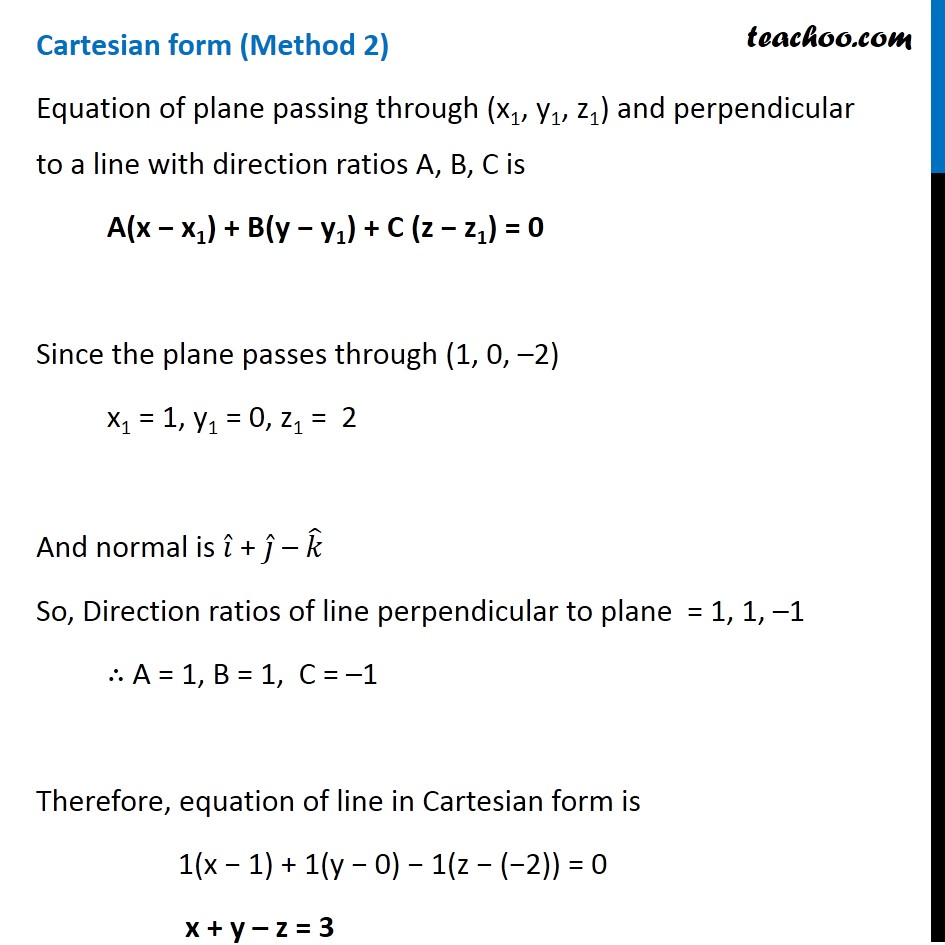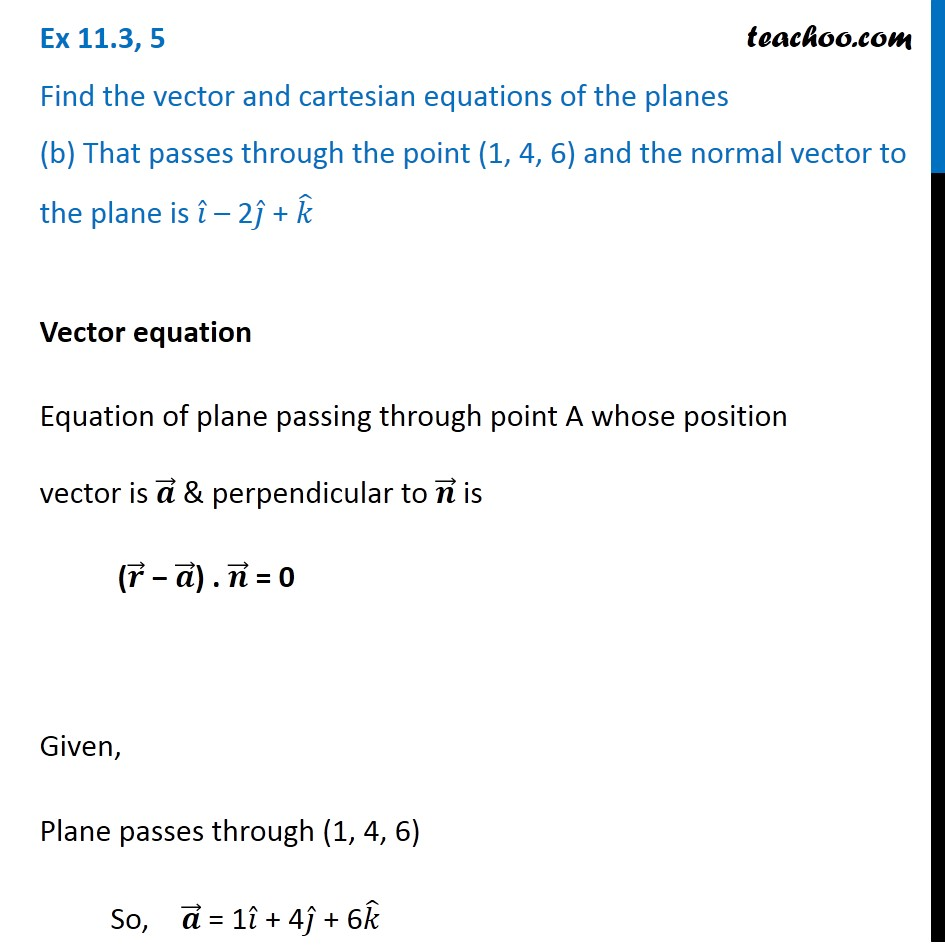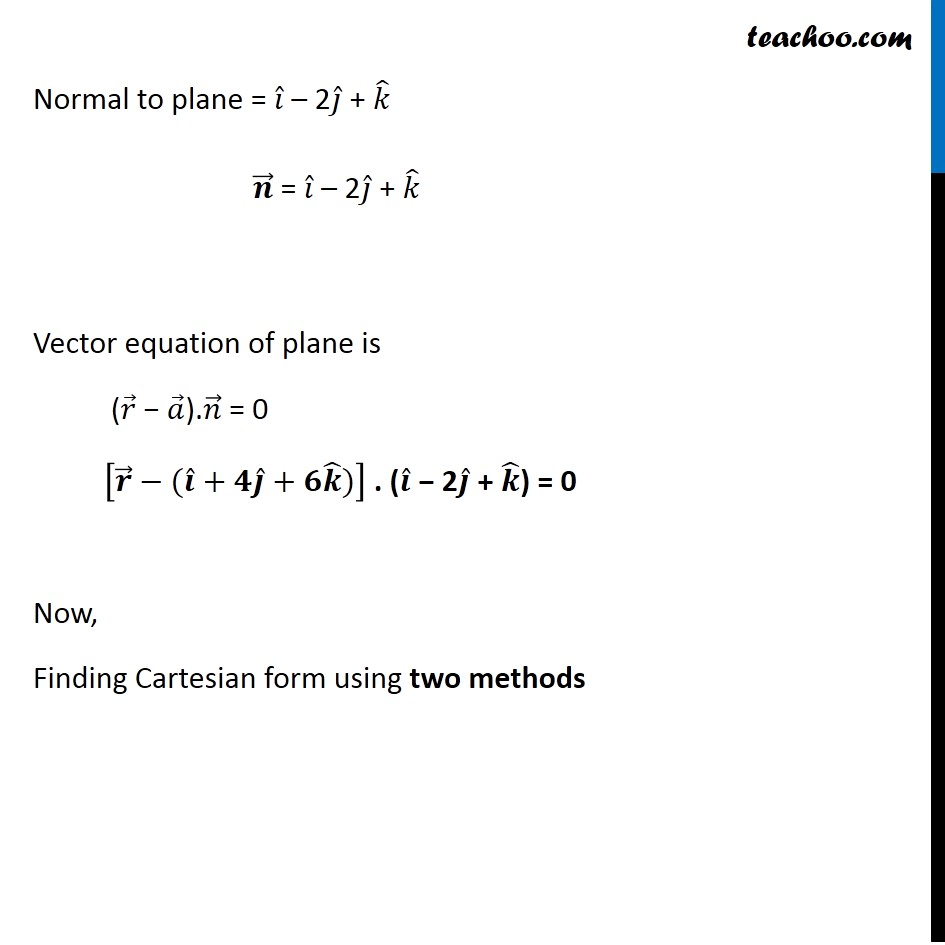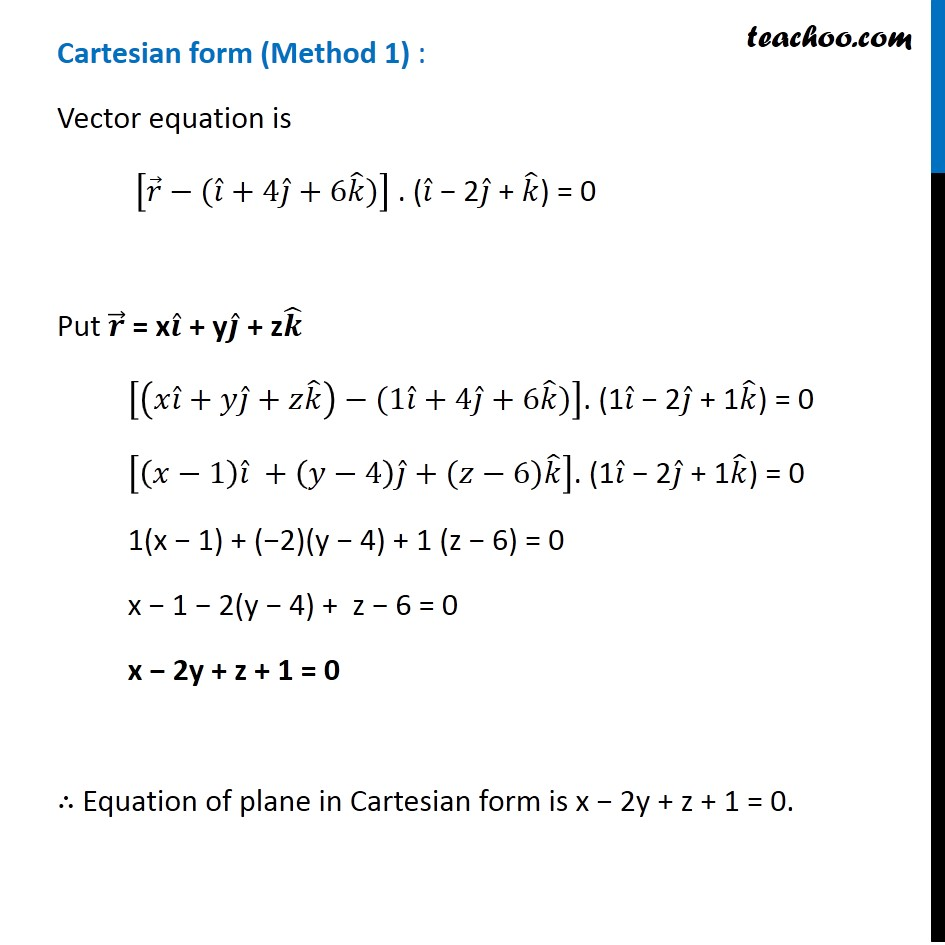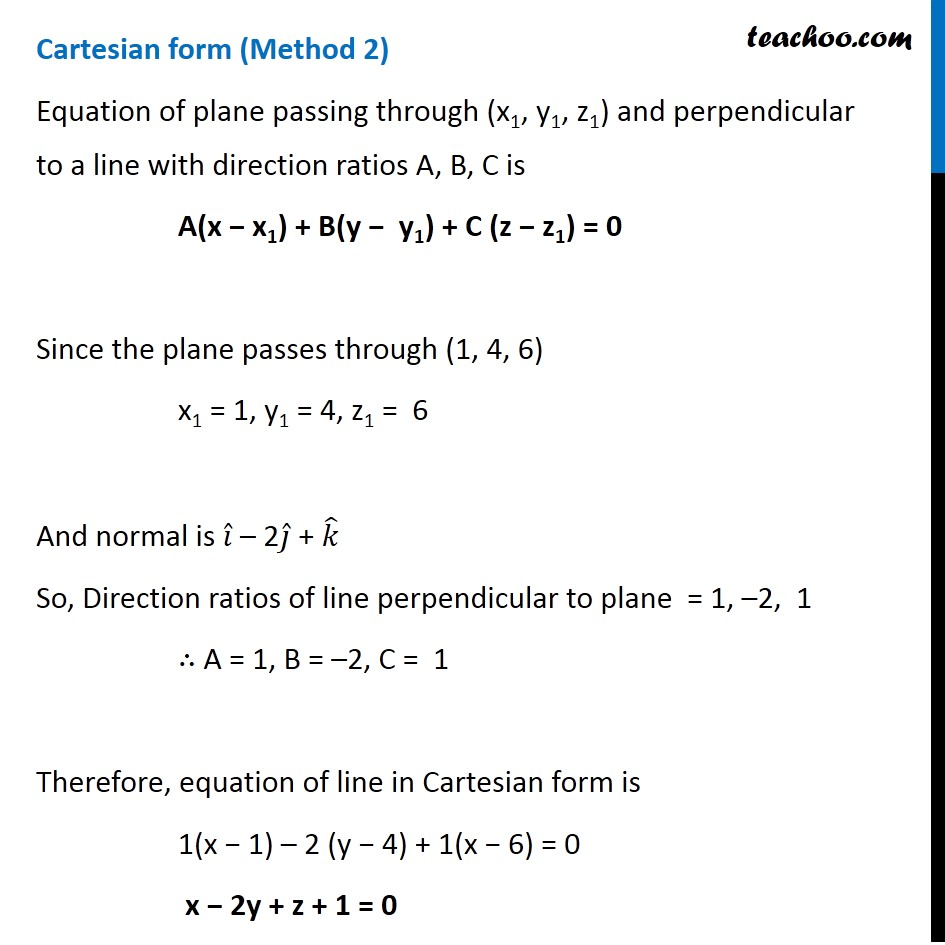Subscribe to our Youtube Channel - https://you.tube/teachoo

1. Chapter 11 Class 12 Three Dimensional Geometry
2. Serial order wise
3. Ex 11.3

Transcript

Ex 11.3, 5 (Introduction) Find the vector and cartesian equations of the planes (a) that passes through the point (1, 0, –2) and the normal to the plane is 𝑖 ̂ + 𝑗 ̂ − 𝑘 ̂.Vector equation of a plane passing through a point (x1, y1, z1) and perpendicular to a line with direction ratios A, B, C is [𝑟 ⃗ −(𝑥1𝑖 ̂+𝑦1𝑗 ̂+𝑧1𝑘 ̂)]. (A𝑖 ̂ + B𝑗 ̂ + C𝑘 ̂) = 0 or (𝑟 ⃗ − 𝑎 ⃗).𝑛 ⃗ = 0 ("A" 𝑃) ⃗ is perpendicular to "n" ⃗ So, ("A" P) ⃗ . "n" ⃗ = 0 ("r" ⃗ − "a" ⃗)."n" ⃗ = 0 Ex 11.3, 5 Find the vector and Cartesian equations of the planes (a) that passes through the point (1, 0, – 2) and the normal to the plane is 𝑖 ̂ + 𝑗 ̂ − 𝑘 ̂.Vector equation Equation of plane passing through point A whose position vector is 𝒂 ⃗ & perpendicular to 𝒏 ⃗ is (𝒓 ⃗ − 𝒂 ⃗) . 𝒏 ⃗ = 0 Given Plane passes through (1, 0, −2) So, 𝒂 ⃗ = 1𝑖 ̂ + 0𝑗 ̂ – 2𝑘 ̂ Normal to plane = 𝑖 ̂ + 𝑗 ̂ – 𝑘 ̂ 𝒏 ⃗ = 𝑖 ̂ + 𝑗 ̂ − 𝑘 ̂ Vector equation of plane is (𝒓 ⃗ − 𝒂 ⃗) . 𝒏 ⃗ = 0 [𝑟 ⃗−("1" 𝑖 ̂+"0" 𝑗 ̂−"2" 𝑘 ̂)].(𝑖 ̂ + 𝑗 ̂ − 𝑘 ̂) = 0 [𝒓 ⃗−(𝒊 ̂−"2" 𝒌 ̂)] . (𝒊 ̂+𝒋 ̂−𝒌 ̂) = 0 Now, Finding Cartesian form using two methods Cartesian form (Method 1) Vector equation is [𝑟 ⃗−(𝑖 ̂−"2" 𝑘 ̂)] . (𝑖 ̂+𝑗 ̂−𝑘 ̂) = 0 Put 𝒓 ⃗ = x𝒊 ̂ + y𝒋 ̂ + z𝒌 ̂ [("x" 𝑖 ̂+"y" 𝑗 ̂+"z" 𝑘 ̂ )−("1" 𝑖 ̂+"0" 𝑗 ̂−"2" 𝑘 ̂)].(1𝑖 ̂ + 1𝑗 ̂ – 1𝑘 ̂) = 0 [("x" −1) 𝑖 ̂+("y" −0) 𝐽 ̂+(𝑧−(−2))𝑘 ̂ ].(1𝑖 ̂ + 1𝑗 ̂ – 1𝑘 ̂) = 0 1(x − 1) + 1(y − 0) – 1 (z + 2) = 0 x − 1 + y – z – 2 = 0 x + y – z = 3 Cartesian form (Method 2) Equation of plane passing through (x1, y1, z1) and perpendicular to a line with direction ratios A, B, C is A(x − x1) + B(y − y1) + C (z − z1) = 0 Since the plane passes through (1, 0, –2) x1 = 1, y1 = 0, z1 = 2 And normal is 𝑖 ̂ + 𝑗 ̂ – 𝑘 ̂ So, Direction ratios of line perpendicular to plane = 1, 1, –1 ∴ A = 1, B = 1, C = –1 Therefore, equation of line in Cartesian form is 1(x − 1) + 1(y − 0) − 1(z − (−2)) = 0 x + y – z = 3 Ex 11.3, 5 Find the vector and cartesian equations of the planes (b) That passes through the point (1, 4, 6) and the normal vector to the plane is 𝑖 ̂ – 2𝑗 ̂ + 𝑘 ̂ Vector equation Equation of plane passing through point A whose position vector is 𝒂 ⃗ & perpendicular to 𝒏 ⃗ is (𝒓 ⃗ − 𝒂 ⃗) . 𝒏 ⃗ = 0 Given, Plane passes through (1, 4, 6) So, 𝒂 ⃗ = 1𝑖 ̂ + 4𝑗 ̂ + 6𝑘 ̂ Normal to plane = 𝑖 ̂ – 2𝑗 ̂ + 𝑘 ̂ 𝒏 ⃗ = 𝑖 ̂ – 2𝑗 ̂ + 𝑘 ̂ Vector equation of plane is (𝑟 ⃗ − 𝑎 ⃗).𝑛 ⃗ = 0 [𝒓 ⃗−(𝒊 ̂+𝟒𝒋 ̂+𝟔𝒌 ̂)] . (𝒊 ̂ − 2𝒋 ̂ + 𝒌 ̂) = 0 Now, Finding Cartesian form using two methods Cartesian form (Method 1) : Vector equation is [𝑟 ⃗−(𝑖 ̂+4𝑗 ̂+6𝑘 ̂)] . (𝑖 ̂ − 2𝑗 ̂ + 𝑘 ̂) = 0 Put 𝒓 ⃗ = x𝒊 ̂ + y𝒋 ̂ + z𝒌 ̂ [(𝑥𝑖 ̂+𝑦𝑗 ̂+𝑧𝑘 ̂ )−(1𝑖 ̂+4𝑗 ̂+6𝑘 ̂)]. (1𝑖 ̂ − 2𝑗 ̂ + 1𝑘 ̂) = 0 [(𝑥−1) 𝑖 ̂ +(𝑦−4) 𝑗 ̂+(𝑧−6)𝑘 ̂ ]. (1𝑖 ̂ − 2𝑗 ̂ + 1𝑘 ̂) = 0 1(x − 1) + (−2)(y − 4) + 1 (z − 6) = 0 x − 1 − 2(y − 4) + z − 6 = 0 x − 2y + z + 1 = 0 ∴ Equation of plane in Cartesian form is x − 2y + z + 1 = 0. Cartesian form (Method 2) Equation of plane passing through (x1, y1, z1) and perpendicular to a line with direction ratios A, B, C is A(x − x1) + B(y − y1) + C (z − z1) = 0 Since the plane passes through (1, 4, 6) x1 = 1, y1 = 4, z1 = 6 And normal is 𝑖 ̂ – 2𝑗 ̂ + 𝑘 ̂ So, Direction ratios of line perpendicular to plane = 1, –2, 1 ∴ A = 1, B = –2, C = 1 Therefore, equation of line in Cartesian form is 1(x − 1) – 2 (y − 4) + 1(x − 6) = 0 x − 2y + z + 1 = 0

Ex 11.3